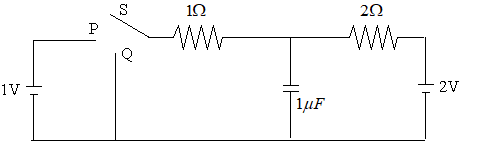Visit the website 123iitjee.manishverma.site for latest posts, courses, admission & more.

###In the circuit shown, the switch S is connected to position P for a long time so that the charge on the capacitor becomes $q_1$ μC. Then S is switched to position Q. After a long time, the charge on the capacitor is $q_2$ μC.

Q.1 The magnitude of $q_1$ is ___ .
Q.2 The magnitude of $q_2$ is ___ .

Solution

The figure below shows the situation when switch S is connected to position P.

No current flows through the capacitor in steady state.

So, $I=\frac {2-1}{1+2}=\frac {1}{3} A$

$V_C =2-2I=2-2\times \frac {1}{3}=\frac {4}{3} V$

$q_1 =CV_C =1\times \frac {4}{3} = \frac {4}{3} \mu C$

Actually, the answer to Q.1 is $q_1 =\frac {4}{3}=1.33$ as the unit is outside.

The figure below shows the situation when switch S is connected to position Q.

Again, no current flows through the capacitor in steady state.

$I=\frac {2}{1+2}=\frac {2}{3} A$

$V_C = \frac {2}{3} \times 1 = \frac {2}{3} V$

$q_2 = 1 \times \frac {2}{3} = \frac {2}{3} \mu C$

Actually, the answer to Q.2 is $q_2 =\frac {2}{3}=0.67$ as the unit is outside.

### Sum of the coefficients in the expansion of $(x+y)^n$ ....

If the sum of the coefficients in the expansion of $(x+y)^n$ is 4096, then the greatest coefficient in the expansion is _ _ _ _ . Solution $C_0 + C_1 + C_2 + C_3 + ......................... + C_n =4096$ $\therefore 2^n = 4096 =2^{12}$ $\Rightarrow n = 12$ Greatest coefficient = ${}^{12}{C_6} = 924$